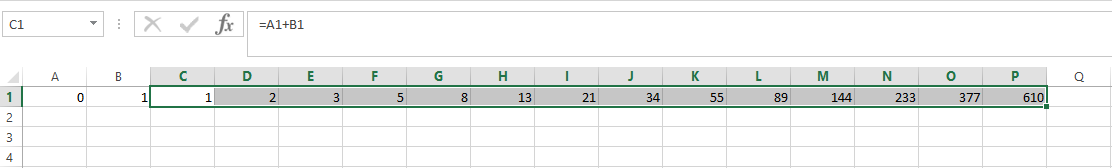### How To Create Fibonacci Series In Excel

Fibonacci numbers form a sequence. After two starting numbers, the next number in the sequence is the sum of the two preceding numbers. The Fibonacci 10 numbers in the Fibonacci sequence are: 0, 1, 2, 3, 5, 8, 13, 21, 44, 65. Here are the steps to create a Fibonacci sequence in Excel:

Click on cell A1 and type the number “0” in it.

Click on cell B1 and type the number “1” in it.

Click on cell C1 and type “=A1+B1” in itTo copy the formula to the adjacent cells, place the mouse pointer on the bottom right side of cell C1. You will see a black plus sign. Drag the plus sign to cell P1. You will see number “612” in cell P1.(optional) To continue the Fibonacci series continue dragging to the right.This will generate Fibonacci sequence automatically.

## Template

```Further reading: